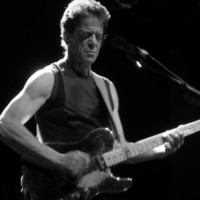música

# Acorde de I'm So Free - Lou Reed```intro   {A}{G}{D}{D}
{A}{G}{D}{D}

Verse   {D}{A/G}{C/G}{G/D}   Yes, I am mother nature's son, and im the
only one
{D}{A/G}{C/G}{G/D}   I do what I want and I want what I see,
you know it happens to me

chorus  {A}{G}{D}{D}         (I'm so free
{C}{G}{D}{D}         I'm so free

Solo    {A}{G}{D}{D}
{A}{G}{D}{D}

Verse   {D}{A/G}{C/G}{G/D}   Oh please, Saint Germaine, I have come
this way
{D}{A/G}{C/G}{G/D}   Do you remember the shape I was in, I had
horns and fins

chorus  {A}{G}{D}{D}         I'm so free
{C}{G}{D}{D}         I'm so free

Verse   {D}{D}{G}{G/D}       Do you remember the silver walks, you
used to shiver, and I used to talk
{D}{D}{G}{G/D}       Then we went down to Times Square, and
http://www.coveralia.com/acordes/i-m-so-free-lou-reed.php
ever since then I've been hangin round
there

chorus  {A}{G}{D}{D}         I'm so free
{C}{G}{D}{D}         I'm so free

Verse   {D}{A/G}{C/G}{G/D}   Yes, I am mother nature's son, and im the
only one
{D}{A/G}{C/G}{G/D}   I do what I want and I want what, and I
want what I see, could only happen to me

chorus  {A}{G}{D}{D}         I'm so free
{C}{G}{D}{D}         I'm so free, Oh-oh

Outro   {G}{D}{G}{D}         oh, I'm so free, oh-oh-oh
{G}{D}{G}{D}         oh, I'm so free, early in the evening
{G}{D}{G}{D}         I'm so free, yeah
{G}{D}{G}{D}         good, I'm so free, when it's ...)
... repeat and fade out

helllllla gooood song

```

Letra subida por: Anónimo

Discos en los que aparece este acorde: Transformer

El acorde de I'm So Free de Lou Reed es una versión de la canción original realizada por colaboradores/usuarios de Coveralia.

¿Has encontrado algún error en esta página? Envíanos tu corrección del acorde

### Zona de usuario

 Recuperar contraseña Regístrate ¿Por qué registrarme?